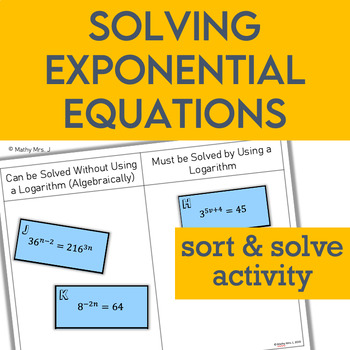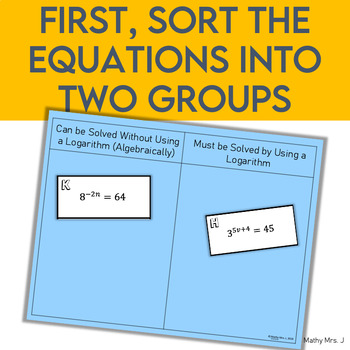# Solving Exponential Equations Card Sort ActivitySubject
Resource Type
File Type

PDF

(1 MB|9 pages)
Product Rating
4.0
(1 Rating)
Standards
Also included in:
1. This bundle includes all my resources for teaching exponential and logarithmic functions. There are activities to use as introductory learning experiences as well as task cards for practice.Thanks for checking out my store, Mathy Mrs. J! Please leave feedback to help me continue to improve my produc
\$13.05
\$9.00
Save \$4.05
• Product Description
• StandardsNEW

This is a great activity to bridge the gap between the two methods of solving exponential equations. If students already know one method this would be a great introduction to learning the other method. Additionally, it could be used as a recap of both methods to solidify student understanding and develop their procedural fluency in solving exponential equations.

Students sort 16 equations into two categories: Equations that can be solved without logarithms (by rewriting with a common base) and equations that can be solved with logarithms. Then they create a mini poster showing how to solve each type of problem

Explain each step in solving a simple equation as following from the equality of numbers asserted at the previous step, starting from the assumption that the original equation has a solution. Construct a viable argument to justify a solution method.
Use the properties of exponents to transform expressions for exponential functions. For example the expression 1.15 to the 𝘵 power can be rewritten as ((1.15 to the 1/12 power) to the 12𝘵 power) is approximately equal to (1.012 to the 12𝘵 power) to reveal the approximate equivalent monthly interest rate if the annual rate is 15%.
Total Pages
9 pages
Included
Teaching Duration
30 minutes
Report this Resource to TpT
Reported resources will be reviewed by our team. Report this resource to let us know if this resource violates TpT’s content guidelines.